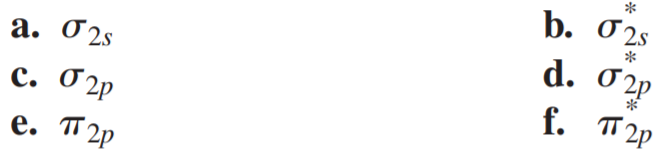×
Get Full Access to Chemistry: A Molecular Approach - 3 Edition - Chapter 10 - Problem 24e
Get Full Access to Chemistry: A Molecular Approach - 3 Edition - Chapter 10 - Problem 24e

×

# Sketch each molecular orbital.ISBN: 9780321809247 1

## Solution for problem 24E Chapter 10

Chemistry: A Molecular Approach | 3rd Edition

• Textbook Solutions
• 2901 Step-by-step solutions solved by professors and subject experts
• Get 24/7 help from StudySoup virtual teaching assistantsChemistry: A Molecular Approach | 3rd Edition

4 5 1 430 Reviews
15
1
Problem 24E

Sketch each molecular orbital.Step-by-Step Solution:

Solution :Step 1 : Here we have to calculate pressure of the gas enclosed in torr for the situations given :(a) The mercury in the arm attached to the gas is 15.4 mm higher than in the one opento the atmosphere; atmospheric pressure is 0.985 atm :Given, height of the mercury = 15.4 mm Atmospheric pressure = 0.985 atm. Now let’s convert 0.985 atm to torr : 1 atm = 760 torr Hence, 0.985 atm in torr is : = 748.6 torr. Therefore, 0.985 atm in torr is 0.684 . Now , let’s determine the pressure of the liquid : 1 mmHg = 1 torrThe mercury in the arm of the manometer is 15.4 mm higher than in the one open toatmosphere, indicating pressure inside the container is lower than the atmospheric pressure. Hence, PgasPatm h = 748.6 torr - 15.4 torr = 733.2 torr.Hence, pressure of the gas in the manometer is 733.2 torr.

Step 2 of 2

##### ISBN: 9780321809247

Unlock Textbook Solution